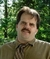## Fixed string length in .NETTags:
2D arrays
Arrays
VB.NET
I am a little stuck. I am reading data from a dataset that contains a string that may contain up to 3 models. Each may be up to 15 char in length each. I get an error when trying to load this data to my array. How can I set it up to load  1-15 into modelA, 16-30 into mobelB and31-45 into modelC?
```        ' get table with the model number
Dim dt As DataTable = dsOrder.Tables(1)
For Each dr As DataRow In dt.Rows

If dr("ModelNumber") > "" Then
Dim Model As String = dr("ModelNumber").ToString
Dim arrModel(3, 2) As String

arrModel(0, 0) = Model.Substring(0, 15)
arrModel(0, 1) = "modelA"
arrModel(1, 0) = Model.Substring(16, 15)
arrModel(1, 1) = "modelB"
arrModel(2, 0) = Model.Substring(31, 15)```
arrModel(2, 1) = "modelC"

Software/Hardware used:
i-series, SQL server 2008

Thanks. We'll let you know when a new response is added.

## Discuss This Question: 5 Replies

Thanks. We'll let you know when a new response is added.
• What is the error you are getting. Logic seems ok
report
•The problem arose when trying to break out second model. That was less than 15.Stating to get it from 16 for a length of 15 and that all 15 were not there gave an indexing error.
report
•Was told to try something like this. It        ' get table with the model numbers
```        Dim dt As DataTable = dsOrder.Tables(1)
For Each dr As DataRow In dt.Rows

If dr("ModelNumber") > "" Then
Dim Model As String = dr("ModelNumber").ToString
Dim arrModel(3, 2) As String

If Model.Length > 15 Then
arrModel(0, 0) = Model.Substring(0, 15)
Else
arrModel(0, 0) = Model.Substring(0, Model.Length)
End If
arrModel(0, 1) = "Model1"

If Model.Length >= 30 Then
arrModel(1, 0) = Model.Substring(16, 15)
Else
If Model.Length > 15 And Model.Length < 30 Then
arrModel(1, 0) = Model.Substring(16, Model.Length - 15)
End If
End If
arrModel(1, 1) = "Model2"

If Model.Length >= 45 Then
arrModel(2, 0) = Model.Substring(31, 15)
Else
If Model.Length > 30 And Model.Length < 45 Then
arrModel(2, 0) = Model.Substring(31, Model.Length - 30)
End If
End If ```
arrModel(2, 1) = "Model3" should compensate for segments that are < 15 in length.

report
•Figured it out. Silly me. Still thinking old RPG and not being able to use an index of 0. Changed it to the right starting indexes and now it works fine.
report
• hahaha oh! it was also not in my mind. Good
report